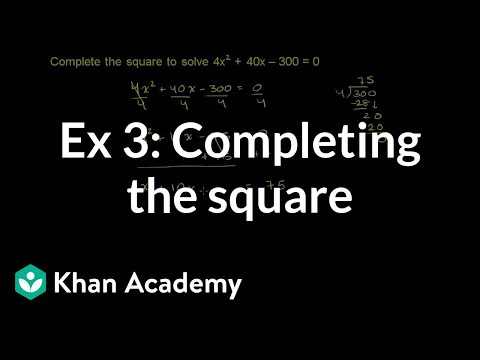# Reasoning with Equations and Inequalities

##By Khan Academy

Sal solves the equation 4x^2+40x-300=0 by completing the square.265# Estimate the Product of Mixed Numbers

##By MathwithMrAlmeida

Use your number sense to estimate what the product will be. A great skill to have to check your work!# Matrices: Basic Matrix Operations (add, subtract, multiply by constant)

##By PatrickJMT

Matrix Operations - Adding, Subtracting, and Multiplying by a constant for matrices is discussed. For more free math videos, visit http://PatrickJMT.com!